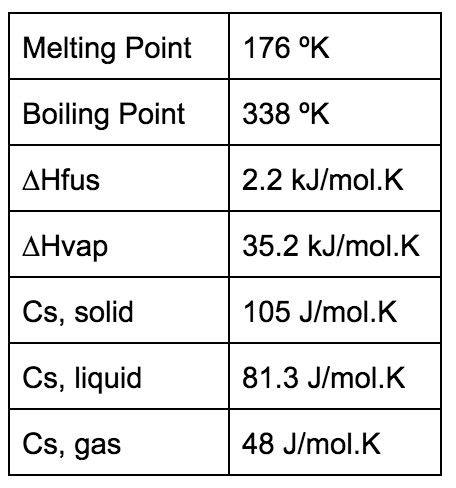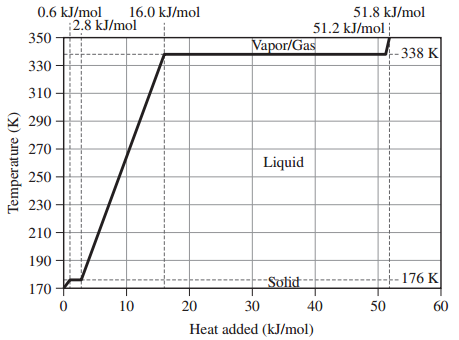# Problem: Assume that the values given here for 1 mole of methanol are constant over the relevant temperature ranges.Given the heating curve for 1 mole of methanol beginning at 170 K and ending at 350 K answer the following questions:What is the value of q gas for methanol?

###### FREE Expert Solution
96% (129 ratings)
###### FREE Expert Solution

q gas → heat absorbed in gas phase.

Given:

n = 1 mol

cgas = 48 J/mol-K

Ti = 338 K

Tf = 350 K

Calculate qgas:

96% (129 ratings)###### Problem Details

Assume that the values given here for 1 mole of methanol are constant over the relevant temperature ranges.Given the heating curve for 1 mole of methanol beginning at 170 K and ending at 350 K answer the following questions:What is the value of q gas for methanol?

Frequently Asked Questions

What scientific concept do you need to know in order to solve this problem?

Our tutors have indicated that to solve this problem you will need to apply the Heating and Cooling Curves concept. You can view video lessons to learn Heating and Cooling Curves. Or if you need more Heating and Cooling Curves practice, you can also practice Heating and Cooling Curves practice problems.

What is the difficulty of this problem?

Our tutors rated the difficulty ofAssume that the values given here for 1 mole of methanol are...as medium difficulty.

How long does this problem take to solve?

Our expert Chemistry tutor, Sabrina took 7 minutes and 14 seconds to solve this problem. You can follow their steps in the video explanation above.

What professor is this problem relevant for?

Based on our data, we think this problem is relevant for Professor Wolfman's class at BC.